# Contribution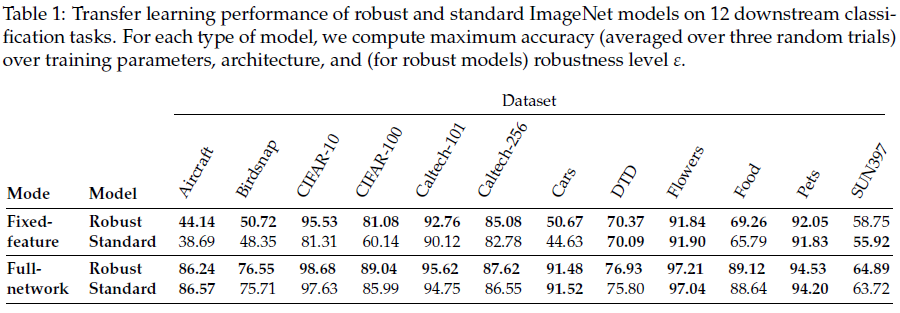Authors identified that adversarial robustness affects transfer learning performance.

Despite being less accurate on ImageNet, adversarially robust neural networks match or improve on the transfer performance of their standard counterparts.

They establish this trend in both “fixed-feature” setting in which one trains a linear classifier on top of feature extracted from a pre-trained network and “full-network” setting in which the pre-trained model is entirely fine-tuned on the relevant downstream task.

# Motivation

## How can we improve transfer learning?

Prior works suggest that accuracy on the source dataset is a strong indicator of performance on downstream tasks.

Still, it is unclear if improving ImageNet accuracy is the only way to improve performance. After all, the behavior of fixed-feature transfer is governed by models’ learned representations, which are not fully described by source-dataset accuracy.

These representations are, in turn, controlled by the priors that we put on them during training.

Adversarial robustness refers to a model’s invariance to small (often imperceptible) perturbations of its inputs.

Robustness is typically induced at training time by replacing the standard empirical risk minimization objective with a robust optimization objective:

$\min _{\theta} \mathbb{E}_{(x, y) \sim D}[\mathcal{L}(x, y ; \theta)] \Longrightarrow \min _{\theta} \mathbb{E}_{(x, y) \sim D}\left[\max _{\|\delta\|_{2} \leq \varepsilon} \mathcal{L}(x+\delta, y ; \theta)\right]$

where $\theta$ is the model parameters, $\mathcal{L}$ is loss function, and $(x, y) \sim D$ are training image-label pairs.

This objective rather than minimizing the loss on the training points, minimizing the worst-case loss over a ball around each training point instead.

## Should adversarial robustness help fixed-feature transfer?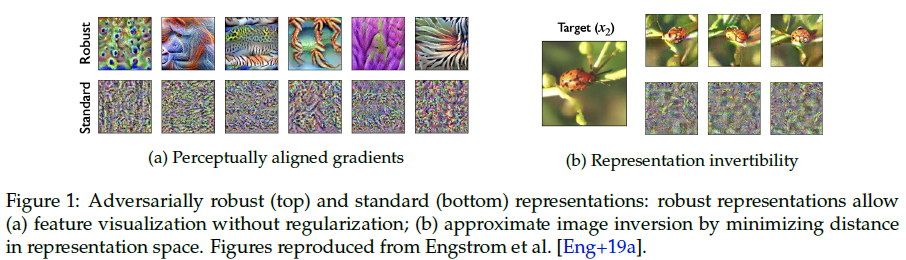On one hand, robustness to adversarial examples may seem somewhat tangential to transfer performance. In fact, adversarially robust models are known to be significantly less accurate than their standard counterparts, suggesting that using adversarially robust feature representations should hurt transfer performance.

On the other hand, recent work has found that the feature representations of robust models carry several advantages over those of standard models. For example, adversarially robust representations have better-behaved gradients and they are approximately invertible meaning that an image can be approximately reconstructed directly from its robust representation. Engstrom et al. hypothesize that the robust training objective leads to feature representations that are more similar to what humans use.

# Adversarial Robustness and Full-Network Fine Tuning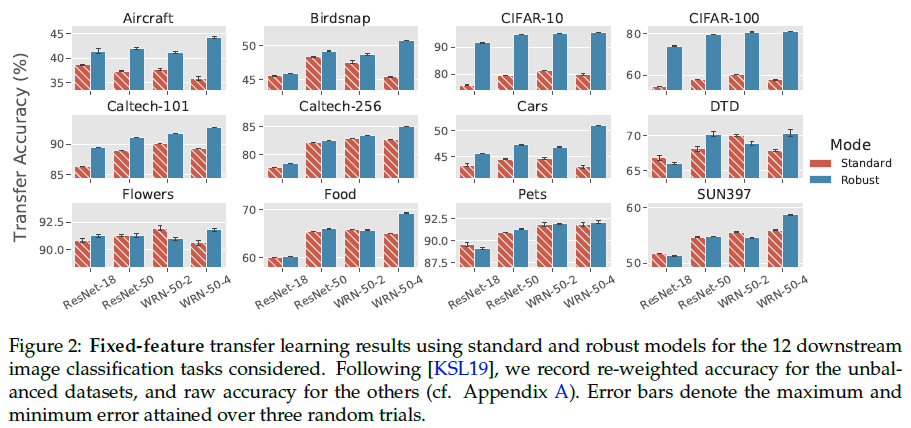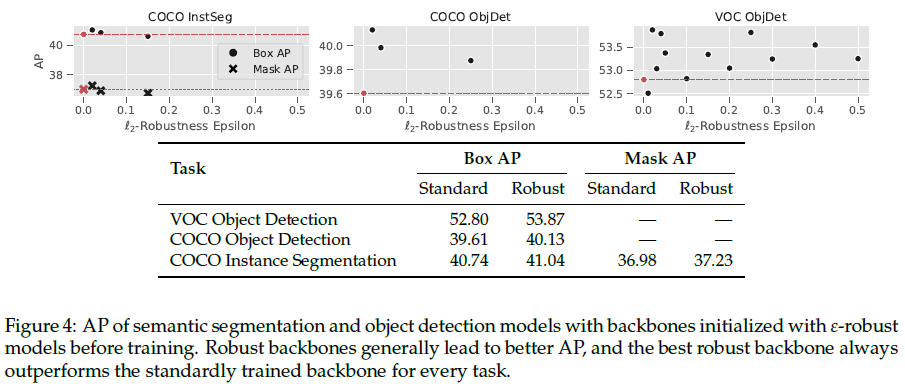Robust models match or improve on the transfer learning performance of standard ones.

# Analysis and Discussion

In this section, we take a closer look at the similarities and differences in transfer learning between robust networks and standard networks.

## ImageNet accuracy and transfer performance

Authors hypothesize that robustness and accuracy have effects which is counteracting but separate. In other words, higher accuracy with fixed robustness and higher robustness with fixed accuracy both improve transfer learning.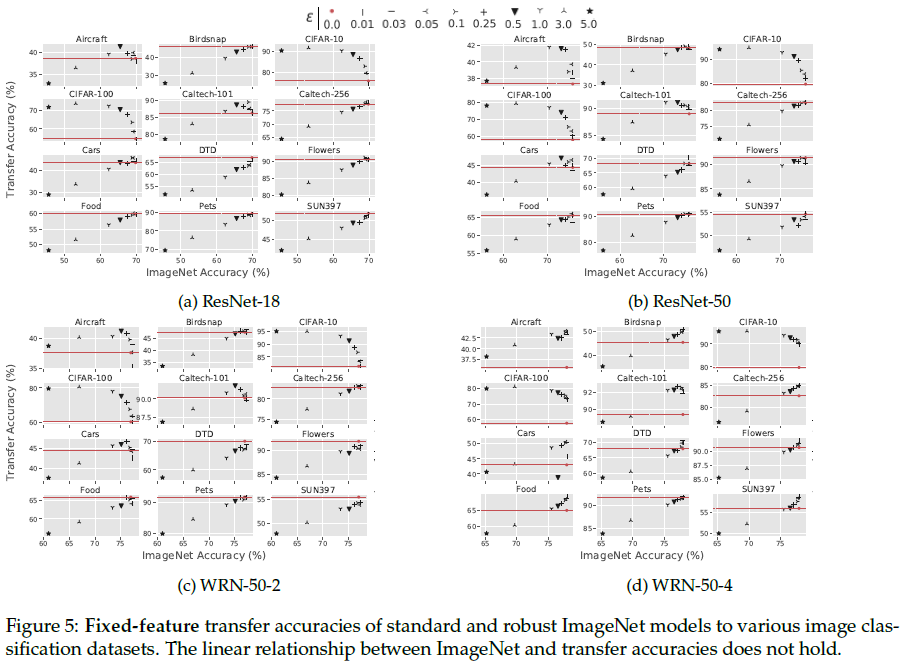To test this hypothesis, they first study the relationship between ImageNet accuracy and transfer accuracy for each of the robust models that they trained. They find that the previously observed linear relationship between accuracy and transfer performance is often violated once the robustness aspect comes into play. (figure 5)Also, they find that when robustness level is held fixed, the accuracy-transfer correlation observed by prior works for standard models holds for robust models too. Table 2 shows that for these models improving ImageNet accuracy improves transfer performance at around the same rate as standard models.

⇒ Transfer learning performance can be further improved by applying known techniques that increase the accuracy of robust models. Accuracy is not sufficient for measuring feature quality or versatility. But we don’t know why robust networks transfer well for now.

## Robust models improve with width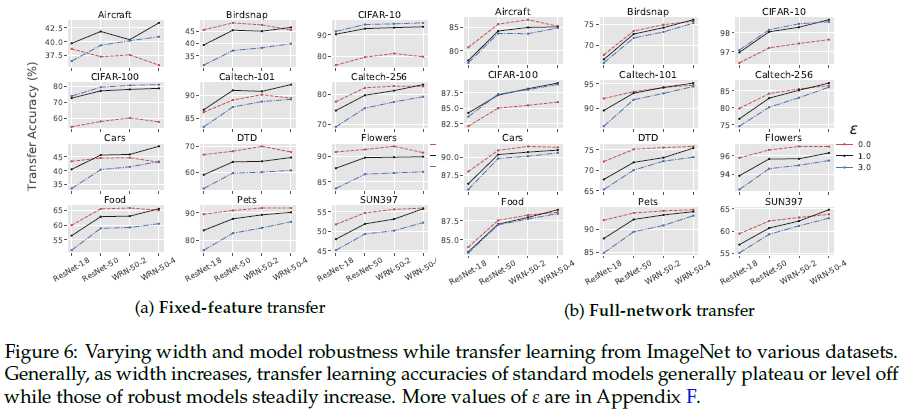## Optimal robustness levels for downstream tasks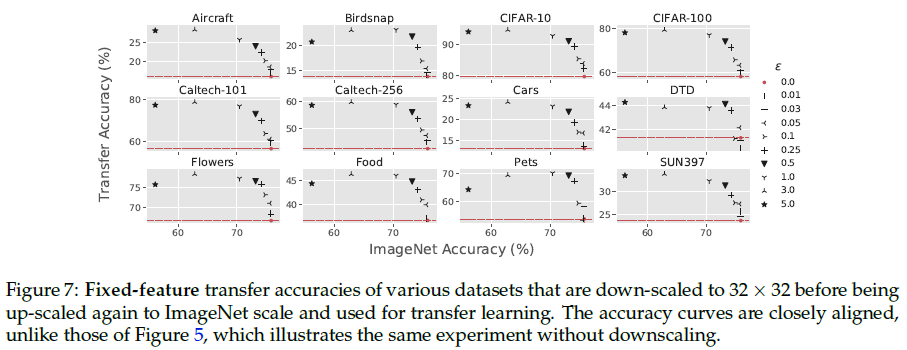Authors observe that although the best robust models often outperform the best standard models, the optimal choice of robustness parameter $\epsilon$ varies widely between datasets. They explain that this variability of optimal choice might relate to dataset granularity. They hypothesize that on datasets where leveraging finer-grained features are necessary, the most effective values of $\epsilon$ will be much smaller than for a dataset where leveraging more coarse-grained features suffices.

Although we lack a quantitative notion of granularity (in reality, features are not simply singular pixels), authors consider image resolution as a crude proxy. They attempt to calibrate the granularities of the 12 image classification datasets used in this work, by first downscaling all the images to the size of CIFAR-10 (32 x 32), and then upscaling them to ImageNet size once more. They then repeat the fixed-feature regression experiments from prior sections, plotting the results in Figure 7. After controlling for original dataset dimension, the datasets’ epsilon vs. transfer accuracy curves all behave almost identically to CIFAR-10 and CIFAR-100 ones.

## Comparing adversarial robustness to texture robustness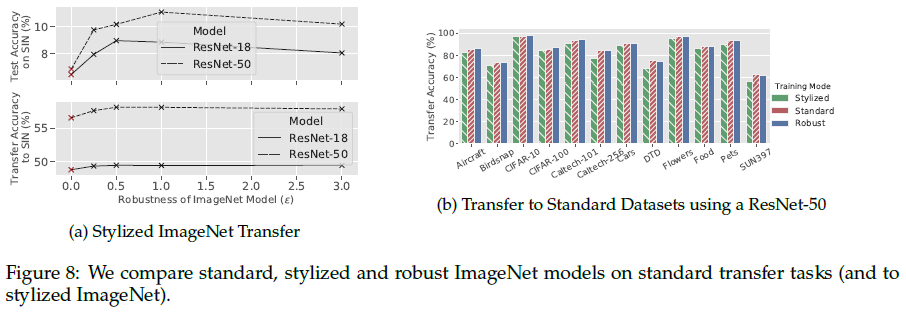Figure 8b shows that transfer learning from adversarially robust models outperforms transfer learning from texture-invariant models on all considered datasets.

Figure 8a top shows that robust models outperform standard imagenet models when evaluated (top) or fine-tuned (bottom) on Stylized-ImageNet.